# Confidence Predictor

A confidence predictor is essentially a prediction algorithm producing prediction regions. In the context of conformal prediction, we assume that Reality outputs successive pairs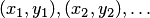called observations. The objects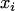are elements of a measurable space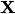and the labels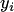are elements of a measurable space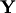.

We call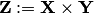the observation space,the significance level, and the complimentary value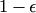the confidence level.

A confidence predictor is a measurable function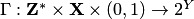(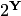is a set of all subsets of) that satisfies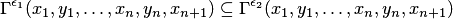for all significance levels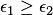, all positive integers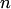, and all incomplete data sequences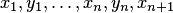. Thus, a confidence predictor is an algorithm that given an incomplete data sequence and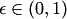(the significance level), outputs a subsetof(the prediction region) so that the condition above is satisfied.